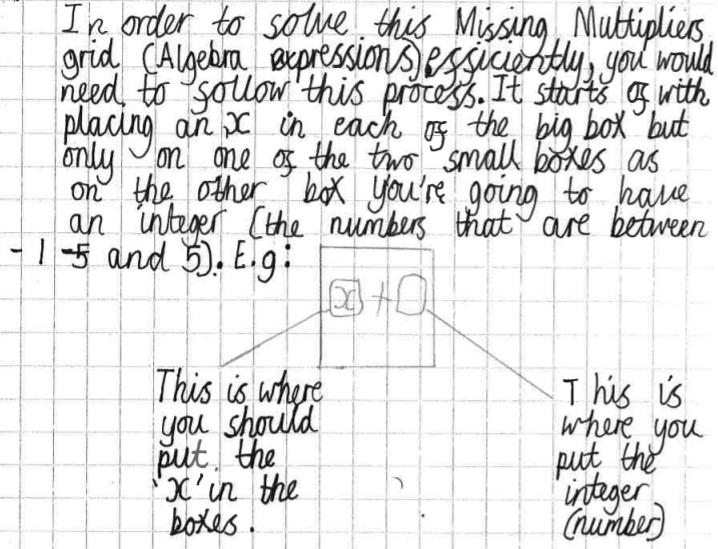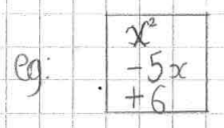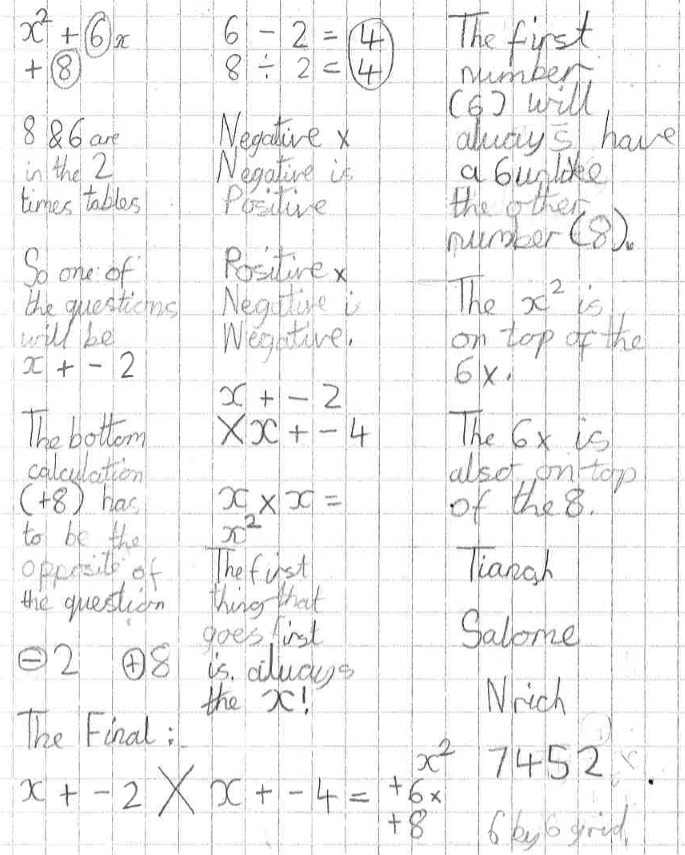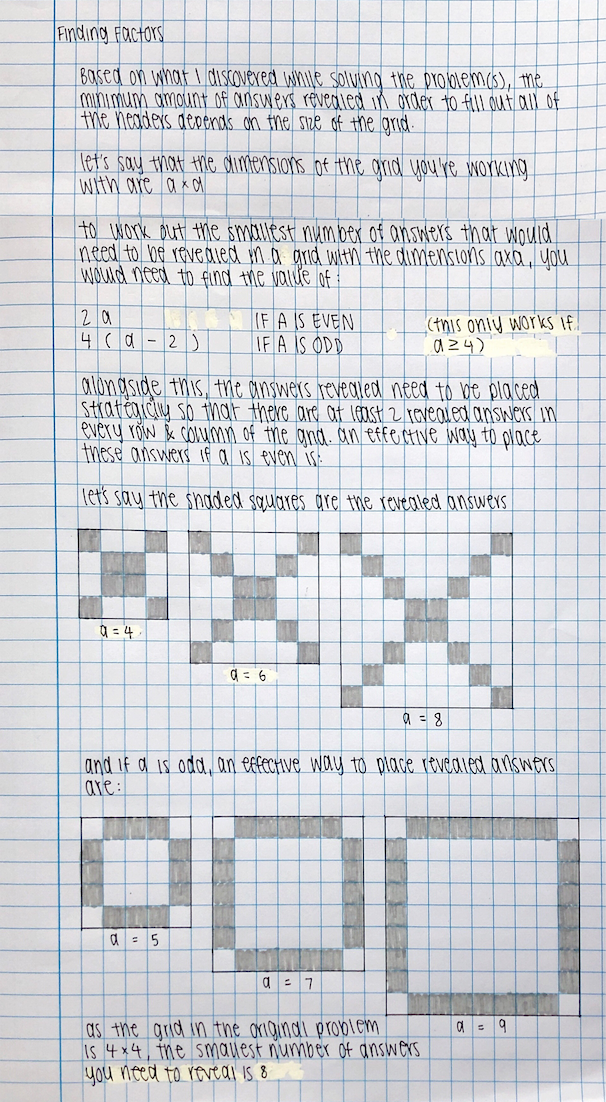#### You may also like### Two Cubes

Two cubes, each with integral side lengths, have a combined volume equal to the total of the lengths of their edges. How big are the cubes? [If you find a result by 'trial and error' you'll need to prove you have found all possible solutions.]### Common Divisor

Find the largest integer which divides every member of the following sequence: 1^5-1, 2^5-2, 3^5-3, ... n^5-n.### Novemberish

a) A four digit number (in base 10) aabb is a perfect square. Discuss ways of systematically finding this number. (b) Prove that 11^{10}-1 is divisible by 100.

# Finding Factors

##### Age 14 to 16 Challenge Level:

Aryan, Zain, Nilukshan, Gharan, Sajuthan, Ibraheem, Tianah and Salome from Northgate Primary in the UK focused on how to find the headings having revealed the answers.
This is the beginning of Sajuthan and Ibraheem's work:Aryan and Zain focused on the signs of the numbers in the answer:If in the [product] the top digit is negative (e.g. $-5$ and the bottom is positive (e.g. $+6$) both numbers that make the [product] are negative.

Nilukshan and Gharan continued this idea:
We found H.C.F. out of the 4 numbers (in this case, the bottom numbers, $+6$) in each row. Then we found if the H.C.F. would be positive or negative. After that we put the negative or positive number on the box of the row.

Tianah and Salome described how to find the two factors from the answer. This is their work. Read down and then across.Tanya from Bangkok Patana School in Thailand thought about how many answers you have to reveal in order to find the solution. This is Tanya's work:It is actually possible to do it by revealing $2a-1$ answers, whether $a$ is even or odd. Can you work out how?